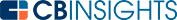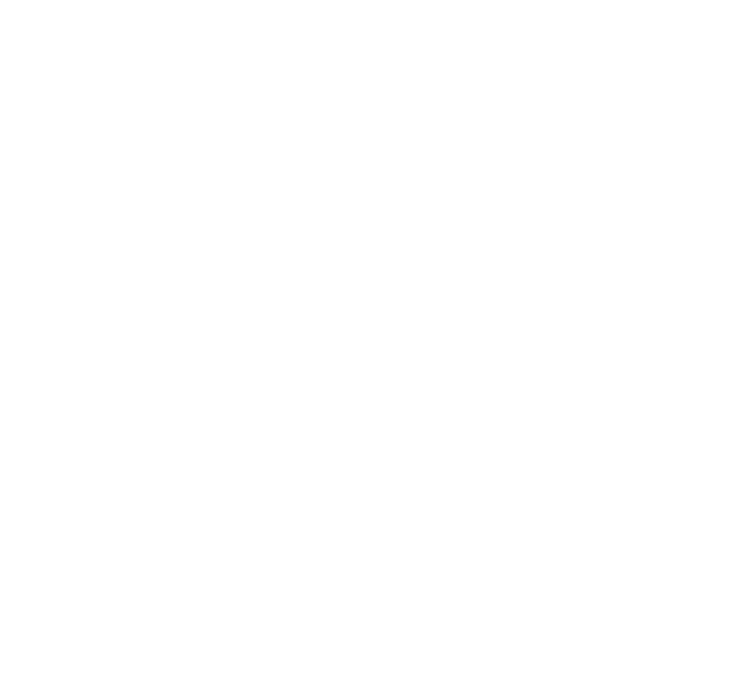# What is the Solow Growth Model?The Solow growth model conveys the idea that developing economies generally experience a higher marginal rate of return on invested capital than developed economies. It postulates the economic production based on capital and labor, which are assumed as substitutable.

Robert M. Solow won a Nobel Prize in 1987 for his contributions to our understanding of factors that influence an economy’s growth. The Solow growth model functions as an extension to the Harrod-Domar model and lays the foundation for the modern theory of economic growth.

## Assumptions of the Solow growth model

The Solow growth model makes the following assumptions:

1. The production function is linearly homogeneous (i.e., the returns to scale are constant). Therefore, when capital and labor increase by 50%, the output should increase by 50% as well.

2. The technology used by firms in an economy remains constant and requires the same proportion of inputs (i.e., capital and labor). The production function, therefore, is represented by the following equation:

Y = aF (K,L)

Here, Y is the output, K is the capital invested, and L is the labor employed.

3. The population growth is constant. For instance, if the population in year 1 is 100 and the growth rate is 5%, the population in years 2 and 3 will be 105 [100(1+0.05)] and 110.25 [105(1+0.05)], respectively.

4. The consumption rate is constant for the entire population. Therefore, the rate of saving remains constant too. The relationship is established through the following consumption equation:

C = Y1 - s

For instance, if the rate of savings is 20% and a person earns 500 units, the person will consume 400 units (500(1-0.2) = 400) and save 100 units.

5. The relationship between the current and future capital stock, the depreciation rate, and capital investment is established with the following equation:

K’ = K(1-d) + I

Here, K’ is the future capital stock, K is the current capital stock, d is the depreciation rate, and I is the capital investment.

## Understanding the Solow growth modelScreenshot via Xplaind.com

Before the point of intersection of the depreciation and investment curve, the investment continues to rise, and vice versa.

This happens because before the point of intersection, the capital increases, consequently pushing up the depreciation. However, beyond the point of intersection, the depreciation exceeds the available investment, consequently shrinking the capital. Therefore, the economy always ends up at the steady state equilibrium.

Essentially, at the steady state equilibrium, all of the capital is used to repair and replace the existing capital, and no new capital is created.

## Implications of the Solow growth model

The Solow growth model implies that an economy will converge to its steady state in the long run and that long-term growth is a result of technological progress.

In its simplest form, the Solow growth model suggests that poor countries grow faster than rich countries as capital accumulates. As they begin to catch up, the growth diminishes. To perpetuate growth, an economy needs technological progress.

For instance, the US, even though it’s a rich country, has been growing for over 2 centuries. More technological progress delivers two-fold benefits. First, it increases the output even while the inputs remain constant. Second, an increase in output spurs an increase in investment.

Several other factors outside of the Solow growth model also influence economic growth, such as political stability and market competitiveness, among others. However, the Solow growth model provides insight into how new ideas and technological advancement can spur sustainable long-term economic growth, other things remaining constant.

You may also like# www.cbinsights.com